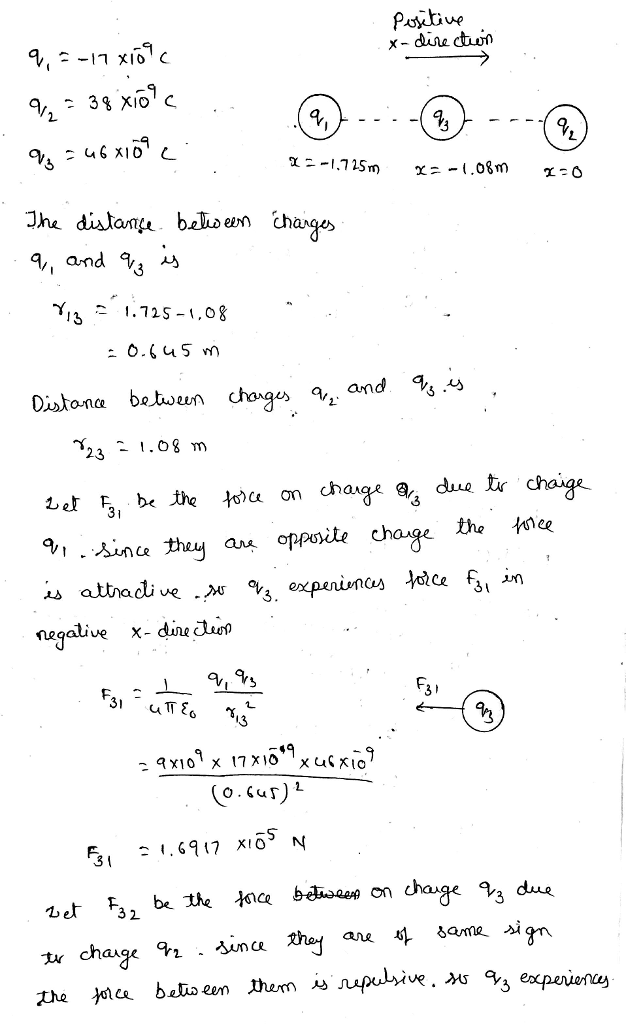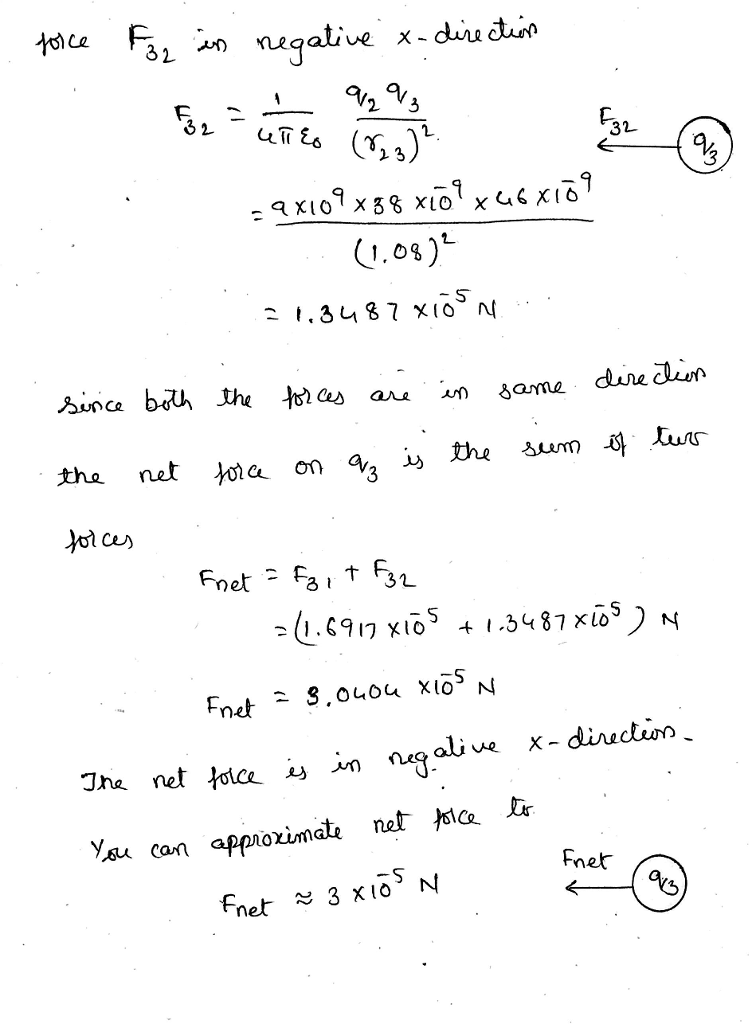Question

# Consider two point charges located on the x axis: one charge, q1 = -17.0 nC ,...

Consider two point charges located on the x axis: one charge, q1 = -17.0 nC , is located at x1 = -1.725 m ; the second charge, q2 = 38.0 nC , is at the origin (x=0.0000). What is the net force exerted by these two charges on a third charge q3 = 46.0 nC placed between q1 and q2 at x3 = -1.080 m ?Share your feedback and doubts by rating and commenting.

#### Earn Coins

Coins can be redeemed for fabulous gifts.

Similar Homework Help Questions
• ### Consider two point charges located on the x axis: one charge, q1 = -10.0 nC ,...

Consider two point charges located on the x axis: one charge, q1 = -10.0 nC , is located at x1 = -1.650 m ;the second charge, q2 = 36.5 nC , is at the origin (x=0.0000). What is the net force exerted by these two charges on a third charge q3 = 52.0 nC placed between q1 and q2 at x3 = -1.205 m ?

• ### Consider two point charges located on the x axis: one charge, q1 = -15.5 nC ,...

Consider two point charges located on the x axis: one charge, q1 = -15.5 nC , is located at x1 = -1.695 m ; the second charge, q2 = 30.5 nC , is at the origin (x=0.0000). What is the net force exerted by these two charges on a third charge q3 = 53.0 nC placed between q1 and q2 at x3 = -1.120 m ? Your answer may be positive or negative, depending on the direction of the force.

• ### Consider two point charges located on the x axis: one charge, q1 = -15.5 nC ,...

Consider two point charges located on the x axis: one charge, q1 = -15.5 nC , is located at x1 = -1.695 m ; the second charge, q2 = 30.5 nC , is at the origin (x=0.0000). What is the net force exerted by these two charges on a third charge q3 = 53.0 nC placed between q1 and q2 at x3 = -1.120 m ? Your answer may be positive or negative, depending on the direction of the force.

• ### Consider two point charges located on the x axis: one charge, q1 = -17.0 nC ,...

Consider two point charges located on the x axis: one charge, q1 = -17.0 nC , is located at x1 = -1.740 m ; the second charge, q2 = 36.5 nC , is at the origin (x = 0). What is (Fnet3)x(Fnet3)x, the x-component of the net force exerted by these two charges on a third charge q3q3q_3 = 50.5 nCnC placed between q1q1 and q2q2 at x3x3x_3 = -1.200 mm?

• ### Two point charges are located on the y-axis as follows: one charge q1 = -1.50 nC...

Two point charges are located on the y-axis as follows: one charge q1 = -1.50 nC located at y= -0.560 m, and a second charge q2 = 3.60 nC at the origin (y=0). What is the magnitude of the total force exerted by these two charges on a third charge q3 = 4.85 nC located at y3 = -0.440 m? What is the direction of the total force?

• ### What is the net force exerted by these two charges on a third charge q3 =...

What is the net force exerted by these two charges on a third charge q3 = 46.5 nC placed between q1 and q2 at x3 = -1.205 m ? Your answer may be positive or negative, depending on the direction of the force. Express your answer numerically in newtons to three significant figures.   Consider two point charges located on the x axis: one charge, q1 = -10.0 nC , is located at x1 = -1.730 m ;the second charge, q2...

• ### Two point charges are placed on the x-axis as follows: charge q1 = 3.98 nC is located at x = 0.196 m, and charge q2 = 4.97 nC is at x =-0.296 m.

Two point charges are placed on the x-axis as follows: charge q1 = 3.98 nC is located at x = 0.196 m, and charge q2 = 4.97 nC is at x =-0.296 m. Part A What is the magnitude of the total force exerted by these two charges on a negative point charge q3 =-6.03 nC that is placed at the origin? Part B What is the direction of the total force exerted by these two charges on a negative point charge q3 =...

• ### Two point charges are placed on the x-axis as follows: Charge q1= 3.90 nC is located...

Two point charges are placed on the x-axis as follows: Charge q1= 3.90 nC is located at x = 0.225m, and charge q2=4.75 nC is at x=-0.295 m. (a) What are the magnitude and direction of the net electric field exerted by these two charges on the origin? (b) What is the voltage (electrostatic potential) at the origin? (c) What is the magnitude and direction of the electrostatic force that acts on a negative point charge q3= -0.610 nC placed...

• ### Two point charges are placed on the x-axis as follows: charge q1 = 4.04 nC is...

Two point charges are placed on the x-axis as follows: charge q1 = 4.04 nC is located at x= 0.203 m , and charge q2 = 5.04 nC is at x= -0.296 m .What is the magnitude of the total force exerted by these two charges on a negative point charge q3 = -6.03 nC that is placed at the origin?

• ### Two point charges are located on the y axis as follows: charge q1 = -2.10 nC...

Two point charges are located on the y axis as follows: charge q1 = -2.10 nC at y1 = -0.650 m , and charge q2 = 2.30 nC at the origin (y = 0). What is the magnitude of the net force exerted by these two charges on a third charge q3 = 5.50 nC located at y3 = 0.200 m ?